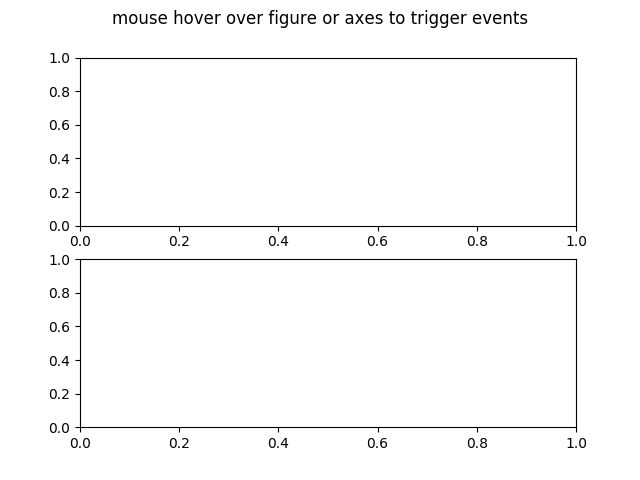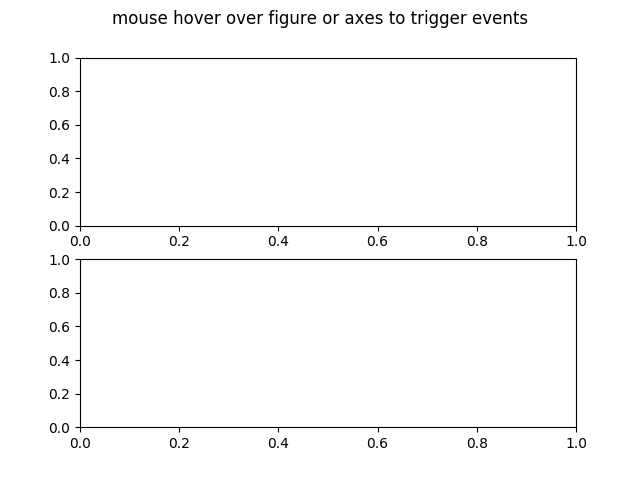Travis-CI:# Figure Axes Enter Leave¶

Illustrate the figure and axes enter and leave events by changing the frame colors on enter and leave

```from __future__ import print_function
import matplotlib.pyplot as plt

def enter_axes(event):
print('enter_axes', event.inaxes)
event.inaxes.patch.set_facecolor('yellow')
event.canvas.draw()

def leave_axes(event):
print('leave_axes', event.inaxes)
event.inaxes.patch.set_facecolor('white')
event.canvas.draw()

def enter_figure(event):
print('enter_figure', event.canvas.figure)
event.canvas.figure.patch.set_facecolor('red')
event.canvas.draw()

def leave_figure(event):
print('leave_figure', event.canvas.figure)
event.canvas.figure.patch.set_facecolor('grey')
event.canvas.draw()
```
```fig1, (ax, ax2) = plt.subplots(2, 1)
fig1.suptitle('mouse hover over figure or axes to trigger events')

fig1.canvas.mpl_connect('figure_enter_event', enter_figure)
fig1.canvas.mpl_connect('figure_leave_event', leave_figure)
fig1.canvas.mpl_connect('axes_enter_event', enter_axes)
fig1.canvas.mpl_connect('axes_leave_event', leave_axes)
``````fig2, (ax, ax2) = plt.subplots(2, 1)
fig2.suptitle('mouse hover over figure or axes to trigger events')

fig2.canvas.mpl_connect('figure_enter_event', enter_figure)
fig2.canvas.mpl_connect('figure_leave_event', leave_figure)
fig2.canvas.mpl_connect('axes_enter_event', enter_axes)
fig2.canvas.mpl_connect('axes_leave_event', leave_axes)

plt.show()
```Total running time of the script: ( 0 minutes 0.066 seconds)

Gallery generated by Sphinx-Gallery